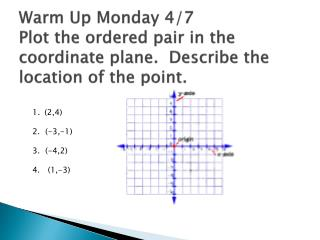DownloadDownload Presentation(2,4) 2. (-3,-1) 3. (-4,2) 4. (1,-3)

# (2,4) 2. (-3,-1) 3. (-4,2) 4. (1,-3)

Download Presentation## (2,4) 2. (-3,-1) 3. (-4,2) 4. (1,-3)

- - - - - - - - - - - - - - - - - - - - - - - - - - - E N D - - - - - - - - - - - - - - - - - - - - - - - - - - -
##### Presentation Transcript

1. Warm Up Monday 4/7Plot the ordered pair in the coordinate plane. Describe the location of the point. (2,4) 2. (-3,-1) 3. (-4,2) 4. (1,-3)

2. Tuesday 4/8 Translation • The vertices of a triangle are j(-2,1), K(-1,3) and L(0,0). Translate the triangle 4 units right (x+4) and 2 units down (y-2). What are the coordinates of the image? Answer: J’ (2,-1), K’(3,1), L’(4,-2)

3. Wednesday 4/9The vertices of a parallelogram are P(0,3), Q (4,3), R (3,1), T(-1,1). Relect the parallelogram in the x-axis. • A. Which coordinate’s sign will change? • B. What are the coordinates of the reflected image? (y) P’ (0,-3), Q’ (4,-3), R’(3,-1), T’ (-1,-1)

4. Thursday 4/10 Mini-assessment 1-3 p. T-401 http://www.bigideasmath.com

5. Friday: 4/11 Watch the next slides and decide if the congruent figure was created by a translation, reflection, rotation, or if it is a dilation in which the figure has changed size. There are 8 slides so number your paper 1-8.

6. See if you can identify the transformation that created the new shapes TRANSLATION

7. See if you can identify the transformation that created the new shapes Where is the line of reflection? REFLECTION

8. See if you can identify the transformation that created the new shapes ROTATION

9. See if you can identify the transformation that created the new shapes DILATION

10. See if you can identify the transformation in these pictures? REFLECTION

11. See if you can identify the transformation in these pictures? ROTATION

12. See if you can identify the transformation in these pictures? REFLECTION

13. See if you can identify the transformation in these pictures? DILATION